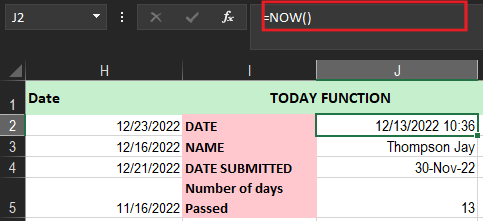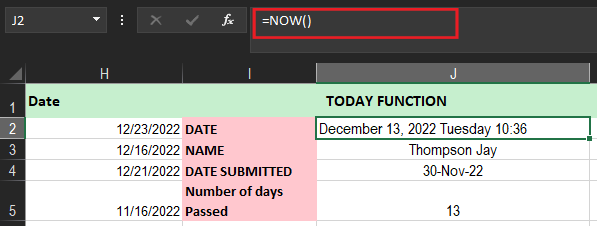# TODAY Function In Excel Examples, Formula & How To Use It

In this tutorial we will learn how to utilize TODAY function in Excel, we will be providing example formulas and ways to do it.

## What is Today Function In Excel

TODAY function is an Excel function wherein returns the current date. Excel stores dates as serial numbers as default therefore it will return a certain value in serial number format. Thus, it must be changed on Date format in order to have a correct format of date.

Apparently, this function can be applied and combined in different functions, thus an aspect of financial analysis for instance.

### Syntax

`=TODAY()`

This syntax does not require arguments but you need to include the empty parenthesis().

Furthermore, this function continuously updates and recalculated every time the worksheet is open and cell value is changed or entered. Thus if the value is changed press F9 in forcing the value to change and update the certain value.

In addition to this, use the keyboard shortcut CRTL+SHIFT; if using static as you enter the current date.

## excel today function Example

Excel TODay function can be joined with other function, so the table below shows how this function interacts and work with other functions.

## How to use the TODAY Function in Excel?

This time the table explains the formula and result of using TODAY function.

## Today function in Excel not Working

If your TODAY function is not working automatically, go to Formulas tab>Calculations Options> and select Automatic. This happens most likely because the recalculation is a turnoff. After, this will update automatically the date.

## Difference between today and now function in excel

The difference between the TODAY and Now function is:

• The TODAY function returns only the date of a user using the function.
• NOW function returns the instant time and date of a user using the function.

## Function finds today’s date in excel

As Today function only return the current date, but not the current time conjunction to date so we can utilize the NOW function for this specific purpose.

1.  Type the formula on cell J5.
2.  Then press CTRL-ENTER to get the current date and time returned as shown below.3. Thus if you want a long date format just, right-click the cell and choose Format Cells.
4.  When the Format Cells Dialog Box appears, select Custom and start customizing the date.
5.  Press Ok and then Excel will show the long date and time as indicated below.## How to add today function in excel to determine number of Days

This time we will determine the number of days of students submitted their requirements, apparently, we can hard code the formula but we want the formula to be updated every time the worksheet is open.

•  Therefore enter the formula in cell J5.
• Upon pressing CTRL-ENTER we get the value 13, which means 13 days have passed since the submitted date of the student at hand and the current date of opening the workbook which was 12 December 2022.
• Secure the number format in cell J5 is General, to see the number of days.

## Conclusion

In conclusion, Today function in excel is crucial when analyzing data which involves current dates and other dates matters which mostly needed in the analysis.

Thank you for reading. Visit our prior tutorials if you have missed some of the functions used in Excel.